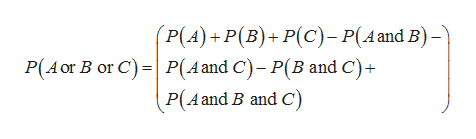# Find P (A or B or C) for the given probabilities. P(A)=0.36, P(B)=0.22, P(C)=0.17P(A and B)=0.11, P(A and C)=0.02, P(B and C)=0.08P(A and B and C)=0.01

Question
38 views

Find P (A or B or C) for the given probabilities.

P(A)=0.36, P(B)=0.22, P(C)=0.17

P(A and B)=0.11, P(A and C)=0.02, P(B and C)=0.08

P(A and B and C)=0.01

check_circle

Step 1

For any three events A, B and C the G...help_outlineImage Transcriptionclose(P(A)+P(B)+P(C)- P(A and B)- P(A and C)- P(B and C) P(Aand B and C) P(Aor B or C) fullscreen

### Want to see the full answer?

See Solution

#### Want to see this answer and more?

Solutions are written by subject experts who are available 24/7. Questions are typically answered within 1 hour.*

See Solution
*Response times may vary by subject and question.
Tagged in

### Other